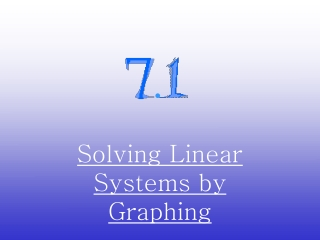DownloadDownload PresentationSolving Linear Systems by Graphing

# Solving Linear Systems by Graphing

Download Presentation## Solving Linear Systems by Graphing

- - - - - - - - - - - - - - - - - - - - - - - - - - - E N D - - - - - - - - - - - - - - - - - - - - - - - - - - -
##### Presentation Transcript

1. . Solving Linear Systems by Graphing

2. Focus 5 Learning Goal –(HS.A-CED.A.3, HS.A-REI.C.5, HS.A-REI.C.6, HS.A-REI.D.11, HS.A-REI.D.12):Students will write, solve and graph linear systems of equations and inequalities.

3. With an equation, any point on the line (x, y) is called a solution to the equation. • With two or more equations, any point that is true for both equations is also a solution to the system.

4. Is (2,-1) a solution to the system? • Check by graphing each equation. Do they cross at (2,-1)? 3x + 2y = 4 -x + 3y = -5 2. Plug the (x,y) values in and see if both equations are true. 3(2) + 2(-1) = 4 6 + (-2) = 4 4 = 4 -2 + 3(-1) = -5 -2 + (-3) = -5 -5 = -5

5. Helpful to rewrite the equations in slope-intercept form. y = -3/2x +2 y = 1/3x – 5/3 Now graph and see where they intersect. Do they cross at (2,-1) ?

6. SOLVE -Graph and give solution then check (plug solution into each equation) y = x + 1 y = -x + 5 Solution (2, 3)

7. Solve: If in standard form, rewrite in slope-intercept form, graph the lines, then plug in to check.

8. y = -2x + 4 2x + y = 4 x – y = 2 y= x - 2 Y y = x + (-2) X 2 -2 Solution: (2,0) y = -2x + 4

9. Check 2x + y = 4x – y = 2 2(2) +0 = 4 2 – 0 = 2 4 =4 2 = 2 Both equations work with the same solution, so (2,0) is the solution to the system.

10. Example 1: If you invest \$9,000 at 5% and 6% interest, and you earn \$510 in total interest, how much did you invest in each account? Equation #1 .05x + .06y = 510 Equation #2x + y = 9,000

11. Solve by graphing (find the x, y-intercepts) When x = 0When y = 0 .05x = 510 x = 10,200 .06y = 510 y = 8,500 -------------------------------------------------------------------------------- x + y = 9,000 y = 9,000 x + y = 9,000 x = 9,000

12. Investment (3,000, 6,000) Solution 1 2 3 4 5 6 7 8 9 10 Thousands at 6% 1 2 3 4 5 6 7 8 9 10 11 Thousands at 5%

13. Graph is upper right quadrant, crossing at (3,000, 6,000) Answer: \$3,000 is invested at 5% and \$6,000 is invested at 6% CHECK ANSWER TO MAKE SURE!!